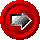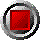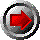HW_BondEuroCall() and HW_Bond_EuroPut() functionsHW_BondEuroCall() and HW_Bond_EuroPut() functions

HW_BondEuroCall(argument list…)

HW_BondEuroPut(argument list…)

These functions return the value of European call and put options on coupon-bearing bonds using the Hull-White analytical model. Note that the market price of the bond is not passed as an argument to the function. Rather, the entire zero curve (including shocks) are passed as arguments, which imply a price for the bond. A function is provided to compute the OAS given a bond price and an unshocked zero curve (see HW_CurveBondPriceOAS). The functions use the following arguments:

 Argument Description Restrictions Valuation_Date valuation date (e.g. today) valid Excel date number Bond_Settlement_At_Expiry bond settlement date when the option expires valid Excel date number >= Valuation_Date Maturity_Date bond maturity date valid Excel date number >= Bond_Settlement_At_Expiry Coupon annual bond coupon in decimal form (e.g. six percent entered as 0.06). For zero coupon (strip) bonds, enter 0. >= 0 Freq number of bond coupons per annum 1, 2, 4, or 12 DCB day count basis 0 = 30/360 (US) 1 = act/act for CAD/US T-Bonds 2 = act/360 3 = act/365 4 = 30/360 (European) Option_Maturity option expiry date valid Excel date number >= Valuation_Date <= Bond_Settlement_At_Expiry Strike option strike per \$100 par > 0 Zero_Dates array of zero coupon curve dates strictly ascending order The first date of this array must be Valuation_Date Zero_Rates array of continuously compounded riskless rates in decimal form (e.g. six percent entered as 0.06) corresponding to Zero_Dates > 0 Short_Rate_Vol annual standard deviation of the short rate of interest, in decimal form > 0 Reversion_Rate mean reversion rate of the short rate of interest, in decimal form >= 0 OAS parallel shift of the zero curve in decimal form Bucket_Start beginning of bucket for zero curve shifts set to 0 if curve shift is not desired Bucket_End end of bucket for zero curve shift >= Bucket_Start Bucket_Shift parallel shift of the zero curve between Bucket_Start and Bucket_End in decimal form set to 0 if curve shock is not desired

© 1995-98 Leap of Faith Research Inc.